Ex 6.1

Chapter 6 Class 12 Application of Derivatives
Serial order wise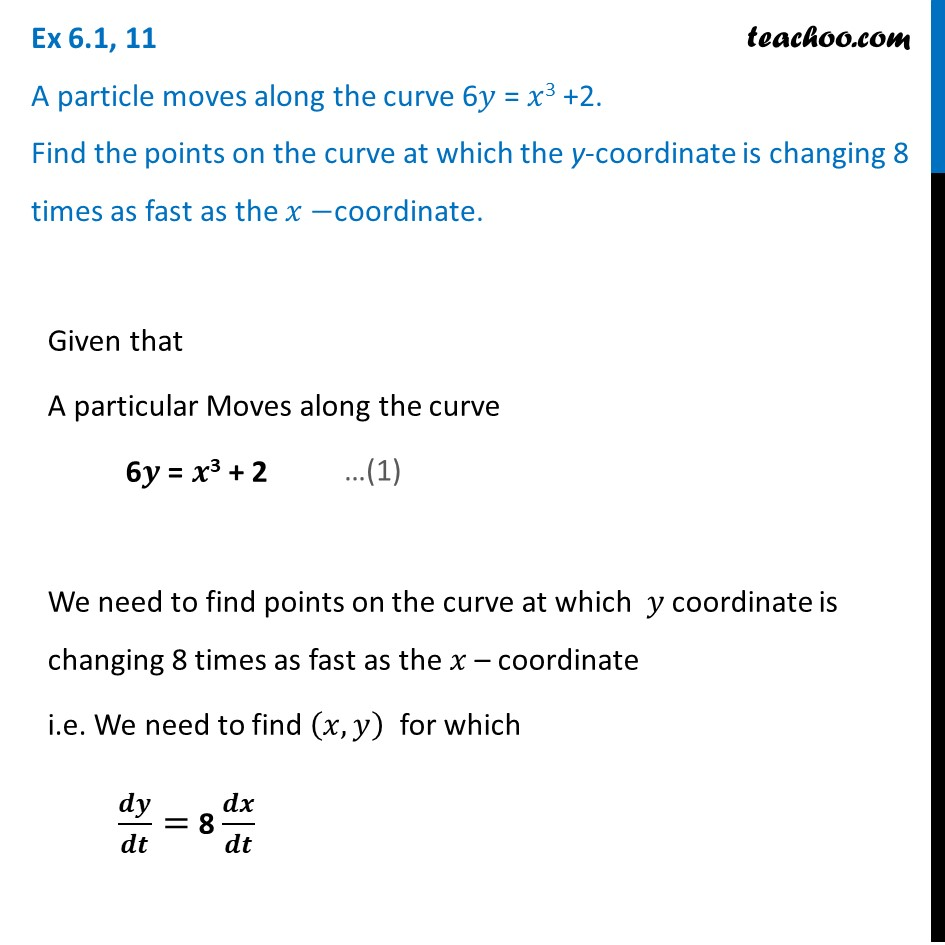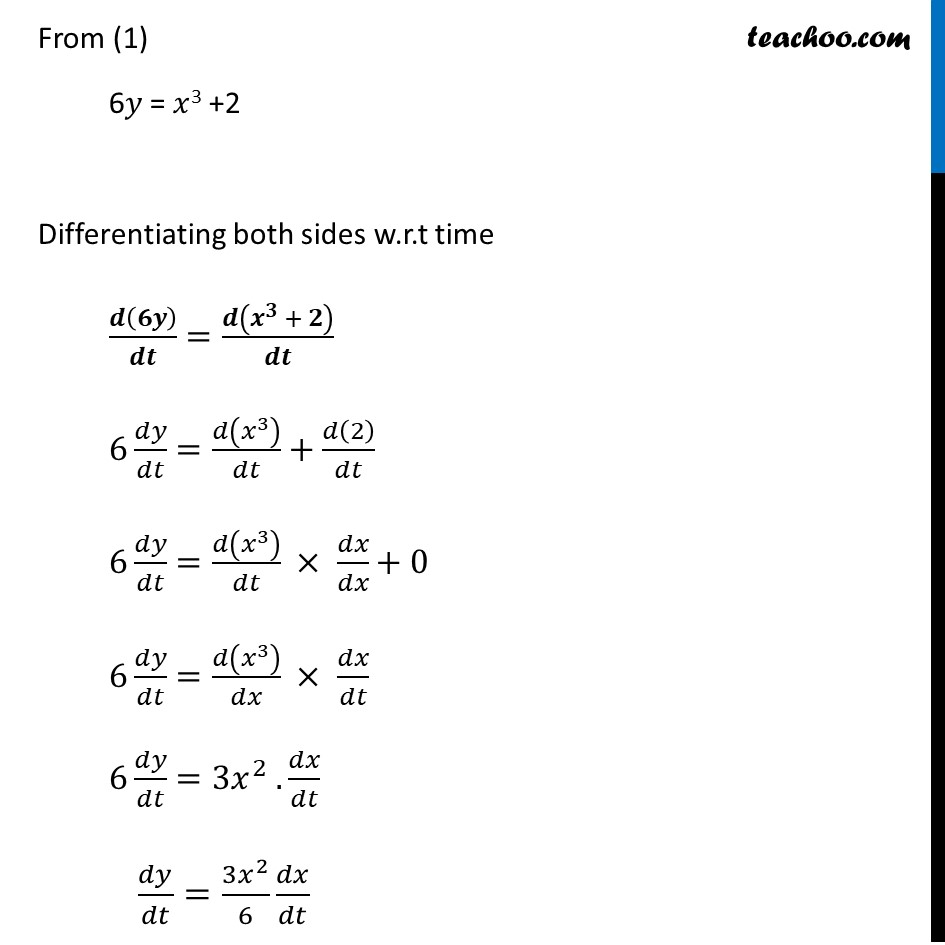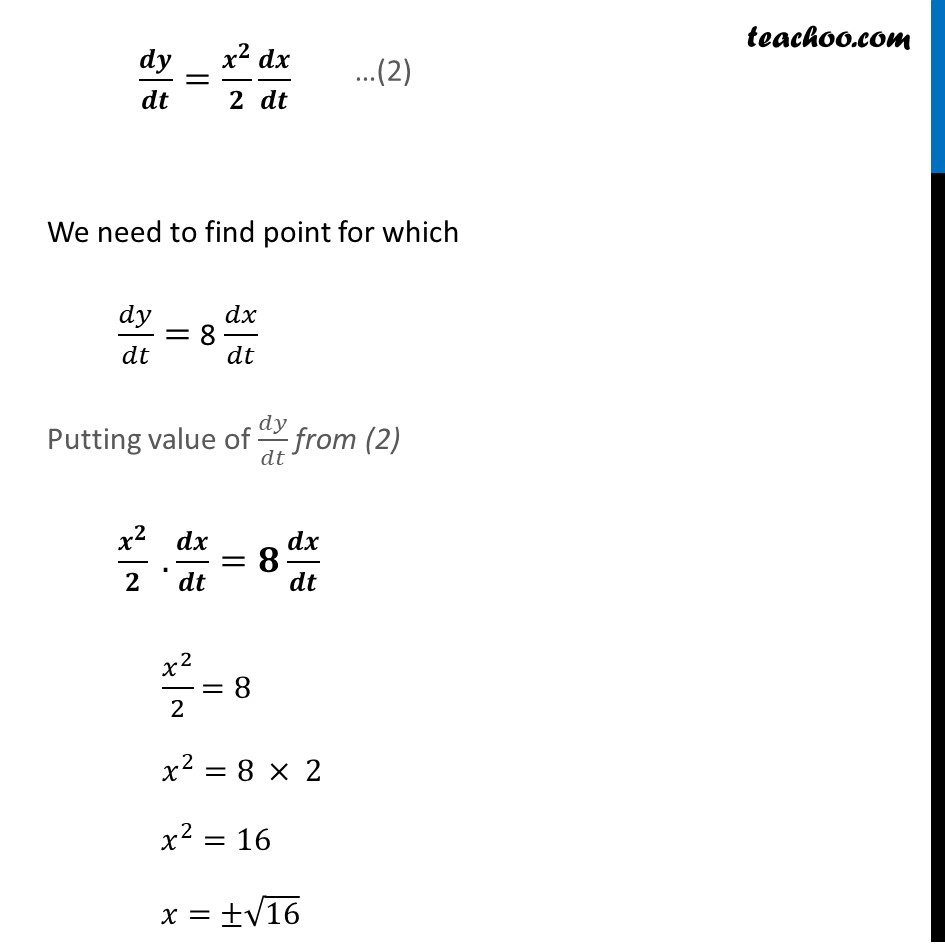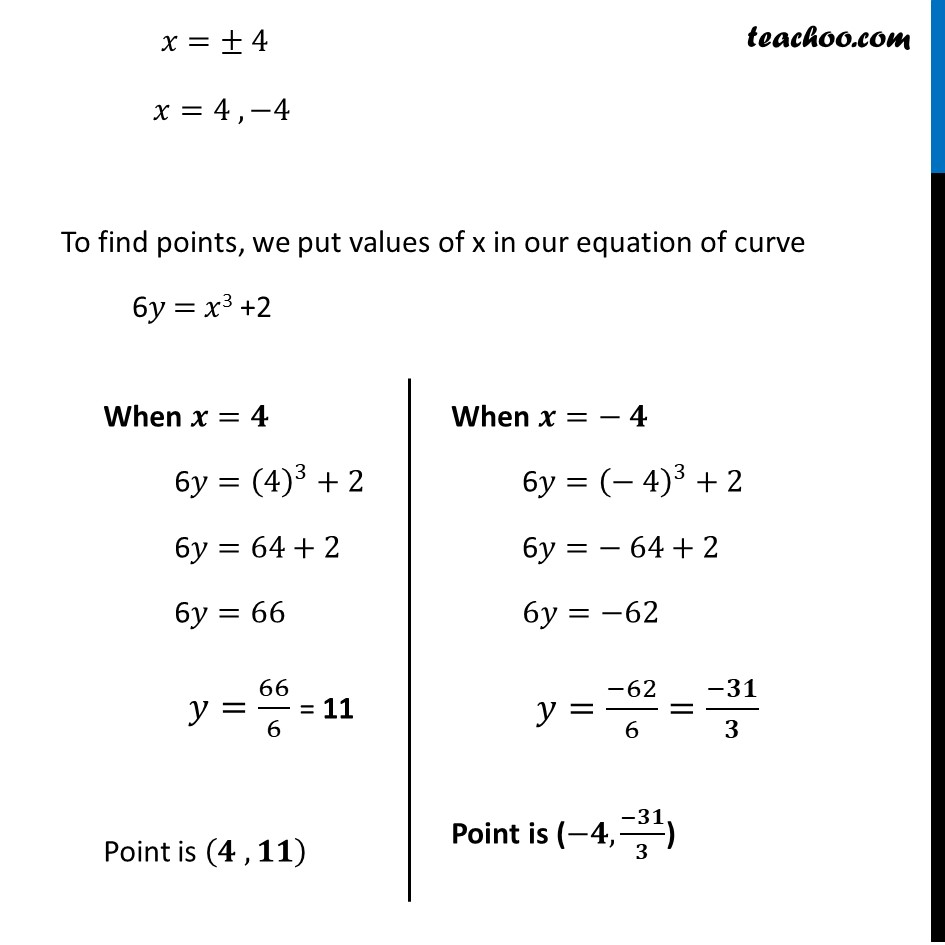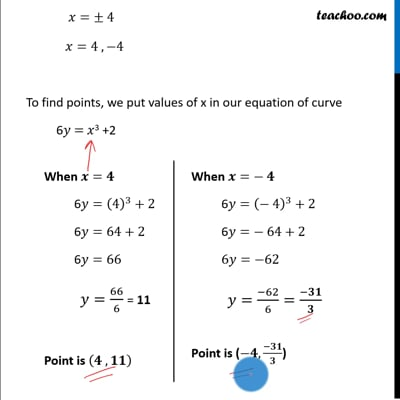This video is only available for Teachoo black users

Maths Crash Course - Live lectures + all videos + Real time Doubt solving!

### Transcript

Ex 6.1, 11 A particle moves along the curve 6𝑦 = 𝑥3 +2. Find the points on the curve at which the y-coordinate is changing 8 times as fast as the 𝑥−coordinate.Given that A particular Moves along the curve 6𝒚 = 𝒙3 + 2 We need to find points on the curve at which 𝑦 coordinate is changing 8 times as fast as the 𝑥 – coordinate i.e. We need to find (𝑥,𝑦) for which 𝒅𝒚/𝒅𝒕= 8 𝒅𝒙/𝒅𝒕 From (1) 6𝑦 = 𝑥3 +2 Differentiating both sides w.r.t time 𝒅(𝟔𝒚)/𝒅𝒕=𝒅(𝒙^𝟑 + 𝟐)/𝒅𝒕 6 𝑑𝑦/𝑑𝑡=𝑑(𝑥^3 )/𝑑𝑡+𝑑(2)/𝑑𝑡 6 𝑑𝑦/𝑑𝑡=𝑑(𝑥^3 )/𝑑𝑡 × 𝑑𝑥/𝑑𝑥+0 6 𝑑𝑦/𝑑𝑡=𝑑(𝑥^3 )/𝑑𝑥 × 𝑑𝑥/𝑑𝑡 6 𝑑𝑦/𝑑𝑡=3𝑥^2 . 𝑑𝑥/𝑑𝑡 𝑑𝑦/𝑑𝑡=(3𝑥^2)/6 𝑑𝑥/𝑑𝑡 𝒅𝒚/𝒅𝒕=𝒙^𝟐/𝟐 𝒅𝒙/𝒅𝒕 We need to find point for which 𝑑𝑦/𝑑𝑡= 8 𝑑𝑥/𝑑𝑡 Putting value of 𝑑𝑦/𝑑𝑡 from (2) 𝒙^𝟐/𝟐 . 𝒅𝒙/𝒅𝒕=𝟖 𝒅𝒙/𝒅𝒕 𝑥^2/2 =8 𝑥^2=8 × 2 𝑥^2=16 𝑥=±√16 𝑥=± 4 𝑥=4 , −4 To find points, we put values of x in our equation of curve 6𝑦 = 𝑥3 +2 When 𝒙=𝟒 6𝑦=(4)^3+2 6𝑦=64+2 6𝑦=66 𝑦=66/6 = 11 Point is (𝟒 , 𝟏𝟏) When 𝒙=− 𝟒 6𝑦=(− 4)^3+2 6𝑦=− 64+2 6𝑦=−62 𝑦=(−62)/6=(−𝟑𝟏)/𝟑 Point is (−𝟒, (−𝟑𝟏)/𝟑)